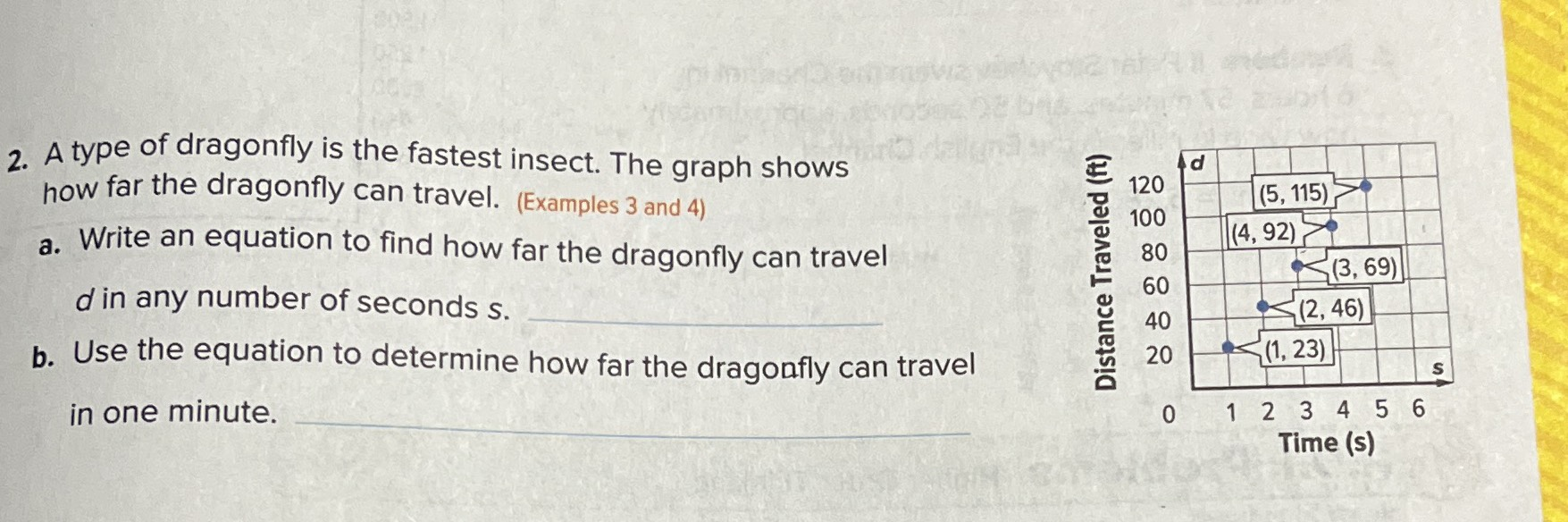### ¿Todavía tienes preguntas de matemáticas?

Pregunte a nuestros tutores expertos
Algebra
Pregunta2. A type of dragonfly is the fastest insect. The graph shows how far the dragonfly can travel. (Examples $$3$$ and 4)

a. Write an equation to find how far the dragonfly can travel $$d$$ in any number of seconds s.

b. Use the equation to determine how far the dragonfly can travel in one minute.

$$d= 23t$$
$$d= 1380t$$